Documentation

Adjust image intensity values or colormap

## Syntax

``J = imadjust(I)``
``````J = imadjust(I,[low_in high_in])``````
``````J = imadjust(I,[low_in high_in],[low_out high_out])``````
``````J = imadjust(I,[low_in high_in],[low_out high_out],gamma)``````
``````J = imadjust(RGB,[low_in high_in],___)``````
``````newmap = imadjust(cmap,[low_in high_in],___)``````

## Description

example

````J = imadjust(I)` maps the intensity values in grayscale image `I` to new values in `J`. By default, `imadjust` saturates the bottom 1% and the top 1% of all pixel values. This operation increases the contrast of the output image `J`.You optionally can perform contrast adjustment using a GPU (requires Parallel Computing Toolbox™).This syntax is equivalent to `imadjust(I,stretchlim(I))`.```
``````J = imadjust(I,[low_in high_in])``` maps intensity values in `I` to new values in `J` such that values between `low_in` and `high_in` map to values between 0 and 1.```

example

``````J = imadjust(I,[low_in high_in],[low_out high_out])``` maps intensity values in `I` to new values in `J` such that values between `low_in` and `high_in` map to values between `low_out` and `high_out`.```
``````J = imadjust(I,[low_in high_in],[low_out high_out],gamma)``` maps intensity values in `I` to new values in `J`, where `gamma` specifies the shape of the curve describing the relationship between the values in `I` and `J`.```

example

``````J = imadjust(RGB,[low_in high_in],___)``` maps the values in truecolor image `RGB` to new values in `J`. You can apply the same mapping or unique mappings for each color channel.```
``````newmap = imadjust(cmap,[low_in high_in],___)``` maps the values in colormap `cmap` to new values in `newmap`. You can apply the same mapping or unique mappings for each color channel.```

## Examples

collapse all

Read a low-contrast grayscale image into the workspace and display it.

```I = imread('pout.tif'); imshow(I)```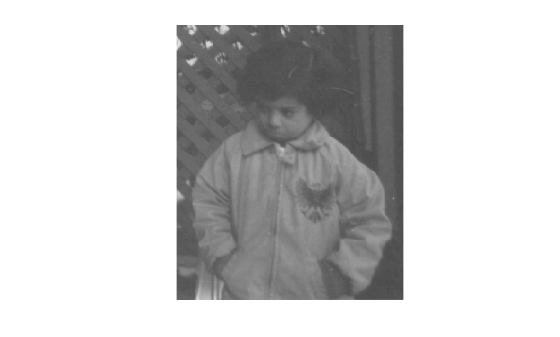Adjust the contrast of the image so that 1% of the data is saturated at low and high intensities, and display it.

```J = imadjust(I); figure imshow(J)```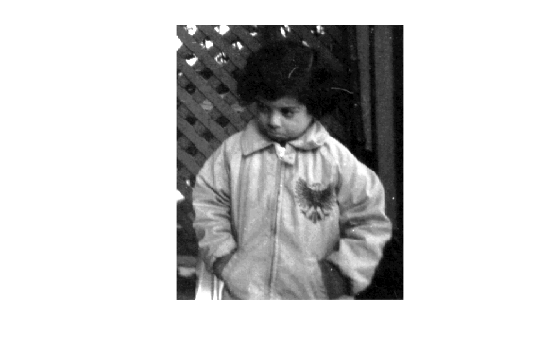Read a low-contrast grayscale image into the workspace and display it.

```I = imread('pout.tif'); imshow(I);```Adjust the contrast of the image, specifying contrast limits.

```K = imadjust(I,[0.3 0.7],[]); figure imshow(K)```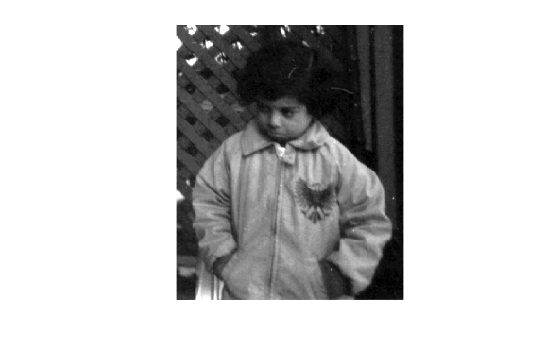Read an RGB image into the workspace and display it.

```RGB = imread('football.jpg'); imshow(RGB)```Adjust the contrast of the RGB image, specifying contrast limits.

```RGB2 = imadjust(RGB,[.2 .3 0; .6 .7 1],[]); figure imshow(RGB2)```Read an image into the workspace, and display it.

```I = imread('pout.tif'); imshow(I)```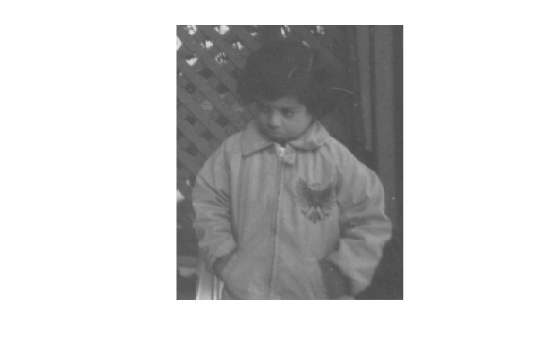Calculate the standard deviation and the image mean for stretching.

```n = 2; Idouble = im2double(I); avg = mean2(Idouble); sigma = std2(Idouble);```

Adjust the contrast based on the standard deviation.

`J = imadjust(I,[avg-n*sigma avg+n*sigma],[]);`

`imshow(J)`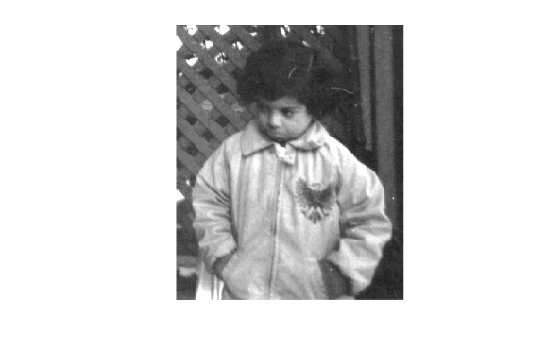## Input Arguments

collapse all

Grayscale image, specified as an m-by-n numeric matrix.

Data Types: `single` | `double` | `int16` | `uint8` | `uint16`

Truecolor image, specified as an m-by-n-by-3 numeric array.

Data Types: `single` | `double` | `int16` | `uint8` | `uint16`

Colormap, specified as a c-by-3 numeric matrix representing c colors.

Data Types: `single` | `double` | `int16` | `uint8` | `uint16`

Contrast limits for input image, specified in one of the following forms:

Contrast Limits

Input TypeValueDescription
grayscale1-by-2 vector of the form ```[low_in high_in]```Specifies the contrast limits in the input grayscale image that you want to map to values in the output image. Values must be in the range `[0 1.0]`. The value `low_in` must be less than the value `high_in`.
RGB or colormap2-by-3 matrix of the form ```[low_RGB_triplet; high_RGB_triplet]```Specifies the contrast limits in the input RGB image or colormap that you want to map to values in the output image or colormap. Each row in the array is an RGB color triplet. Values must be in the range `[0 1]`. The value `low_RGB_triplet` must be less than the value `high_RGB_triplet`.
RGB or colormap1-by-2 vector of the form ```[low_in high_in]```Specifies the contrast limits in the input RGB image that you want to map to values in the output image. Each value must be in the range `[0 1.0]`. The value `low_in` must be less than the value `high_in`. If you specify a 1-by-2 vector with an RGB image or colormap, then `imadjust` applies the same adjustment to each color plane or channel.
all types`[]` If you specify an empty matrix (`[]`), then `imadjust` uses the default limits `[0 1]`.

`imadjust` clips value below `low_in` and above `high_in`: Values below `low_in` map to `low_out` and values above `high_in` map to `high_out`.

Data Types: `single` | `double`

Contrast limits for output image, specified in one of the following forms:

Contrast Limits

Input TypeValueDescription
grayscale1-by-2 vector of the form ```[low_out high_out]```Specifies the contrast limits of the output grayscale image. Each value must be in the range ```[0 1]```.
RGB or colormap2-by-3 matrix of the form ```[low_RGB_triplet; high_RGB_triplet]```Specifies the contrast limits of the output RGB image or colormap. Each row in the array is an RGB color triplet. Values must be in the range ```[0 1]```.
RGB or colormap1-by-2 vector of the form ```[low_out high_out]```Specifies the contrast limits in the output image. Each value must be in the range `[0 1]`. If you specify a 1-by-2 vector with an RGB image or colormap, then `imadjust` applies the same adjustment to each plane or channel.
all types`[]` If you specify an empty matrix (`[]`), then `imadjust` uses the default limits `[0 1]`.

If `high_out` is less than `low_out`, then `imadjust` reverses the output image, as in a photographic negative.

Data Types: `single` | `double`

Shape of curve describing relationship of input and output values, specified as a nonnegative scalar or a 1-by-3 numeric vector.

• If `gamma` is less than 1, then `imadjust` weights the mapping toward higher (brighter) output values.

• If `gamma` is greater than 1, then `imadjust` weights the mapping toward lower (darker) output values.

• If `gamma` is a 1-by-3 vector, then `imadjust` applies a unique gamma to each color component or channel.

• If you omit the argument, then `gamma` defaults to `1` (linear mapping).

Data Types: `double`

## Output Arguments

collapse all

Adjusted image, returned as a grayscale or RGB image. `J` has the same size and class as the input grayscale image `I` or truecolor image `RGB`.

Data Types: `single` | `double` | `int16` | `uint8` | `uint16`

Adjusted colormap, returned as an c-by-3 numeric matrix of the same class as the input colormap, `map`.

Data Types: `single` | `double`